# 2.7.1 Windows 32位系统 Python 2.7 环境 安装 talib 指标库

Author: 小小梦, Created: 2017-02-11 18:01:05, Updated: 2019-08-01 09:20:22

### Windows 32位系统 Python 2.7 环境 安装 talib 指标库

#### 发明者量化 平台中 使用 Python 编写策略时 如果使用 重写过的 TA 库，计算MA 、MACD、EMA 等指标（由于已内置）是不会有任何报错的。

``````import types
def main():
STATE_IDLE = -1
state = STATE_IDLE
initAccount = ext.GetAccount()
while True:
if state == STATE_IDLE :
n = ext.Cross(FastPeriod,SlowPeriod) # 指标交叉函数
if abs(n) >= EnterPeriod :
opAmount = _N(initAccount.Stocks * PositionRatio,3)
Dict = ext.Buy(opAmount) if n > 0 else ext.Sell(opAmount)
if Dict :
opAmount = Dict['amount']
state = PD_LONG if n > 0 else PD_SHORT
Log("开仓详情",Dict,"交叉周期",n)
else:
n = ext.Cross(ExitFastPeriod,ExitSlowPeriod) # 指标交叉函数
if abs(n) >= ExitPeriod and ((state == PD_LONG and n < 0) or (state == PD_SHORT and n > 0)) :
nowAccount = ext.GetAccount()
Dict2 = ext.Sell(nowAccount.Stocks - initAccount.Stocks) if state == PD_LONG else ext.Buy(initAccount.Stocks - nowAccount.Stocks)
state = STATE_IDLE
nowAccount = ext.GetAccount()
LogProfit(nowAccount.Balance - initAccount.Balance,'钱：',nowAccount.Balance,'币：',nowAccount.Stocks,'平仓详情：',Dict2,'交叉周期：',n)
Sleep(Interval * 1000)

``````

#### 如果改一下代码。

``````import types
import talib  # 改动  引用 talib 库
def main():
STATE_IDLE = -1
state = STATE_IDLE
initAccount = ext.GetAccount()
while True:
records = exchange.GetRecords()
ma = talib.MA(records.Close)       # 改动 ，调用 talib 库的 MA 函数 即 均线指标计算
LogStatus("均值" + str(ma))
if state == STATE_IDLE :
n = ext.Cross(FastPeriod,SlowPeriod) # 指标交叉函数
if abs(n) >= EnterPeriod :
opAmount = _N(initAccount.Stocks * PositionRatio,3)
Dict = ext.Buy(opAmount) if n > 0 else ext.Sell(opAmount)
if Dict :
opAmount = Dict['amount']
state = PD_LONG if n > 0 else PD_SHORT
Log("开仓详情",Dict,"交叉周期",n)
else:
n = ext.Cross(ExitFastPeriod,ExitSlowPeriod) # 指标交叉函数
if abs(n) >= ExitPeriod and ((state == PD_LONG and n < 0) or (state == PD_SHORT and n > 0)) :
nowAccount = ext.GetAccount()
Dict2 = ext.Sell(nowAccount.Stocks - initAccount.Stocks) if state == PD_LONG else ext.Buy(initAccount.Stocks - nowAccount.Stocks)
state = STATE_IDLE
nowAccount = ext.GetAccount()
LogProfit(nowAccount.Balance - initAccount.Balance,'钱：',nowAccount.Balance,'币：',nowAccount.Stocks,'平仓详情：',Dict2,'交叉周期：',n)
Sleep(Interval * 1000)

``````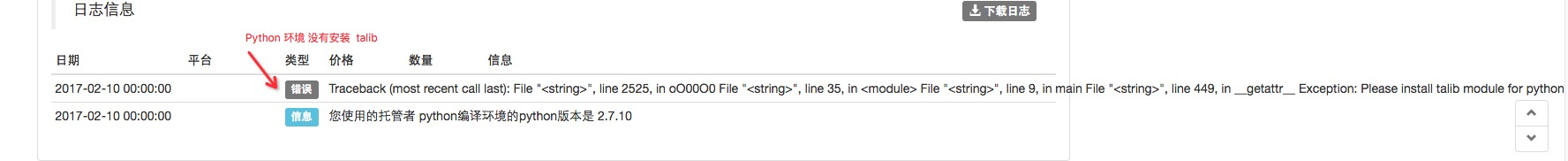• #### 1、准备 下载 安装好 Python 2.7 (win32)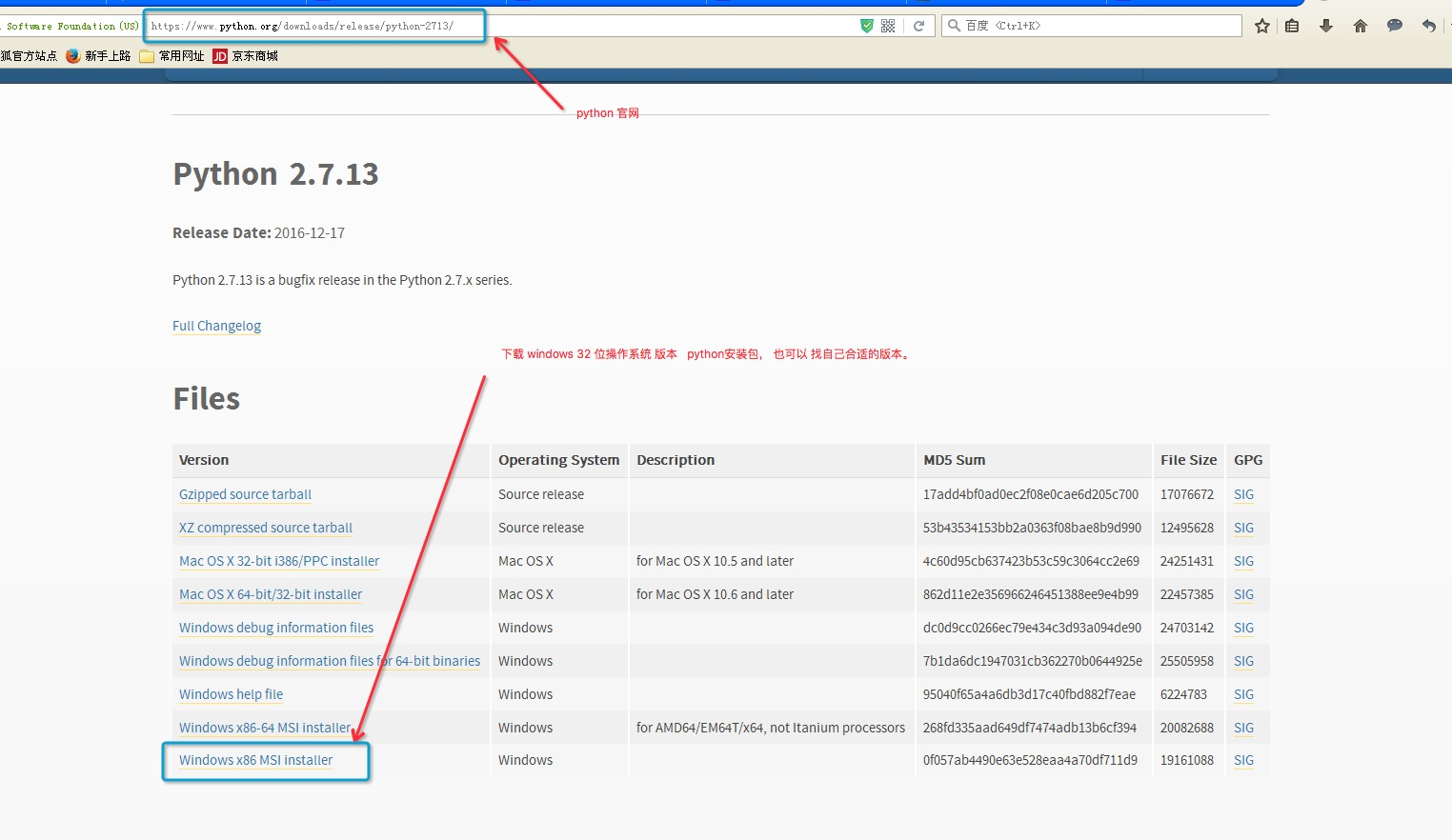注意 win32 版本 Python 2.7 如图 下载安装包。

安装时 注意 选择上自动配置 环境变量的选项， pip 组件已经默认安装。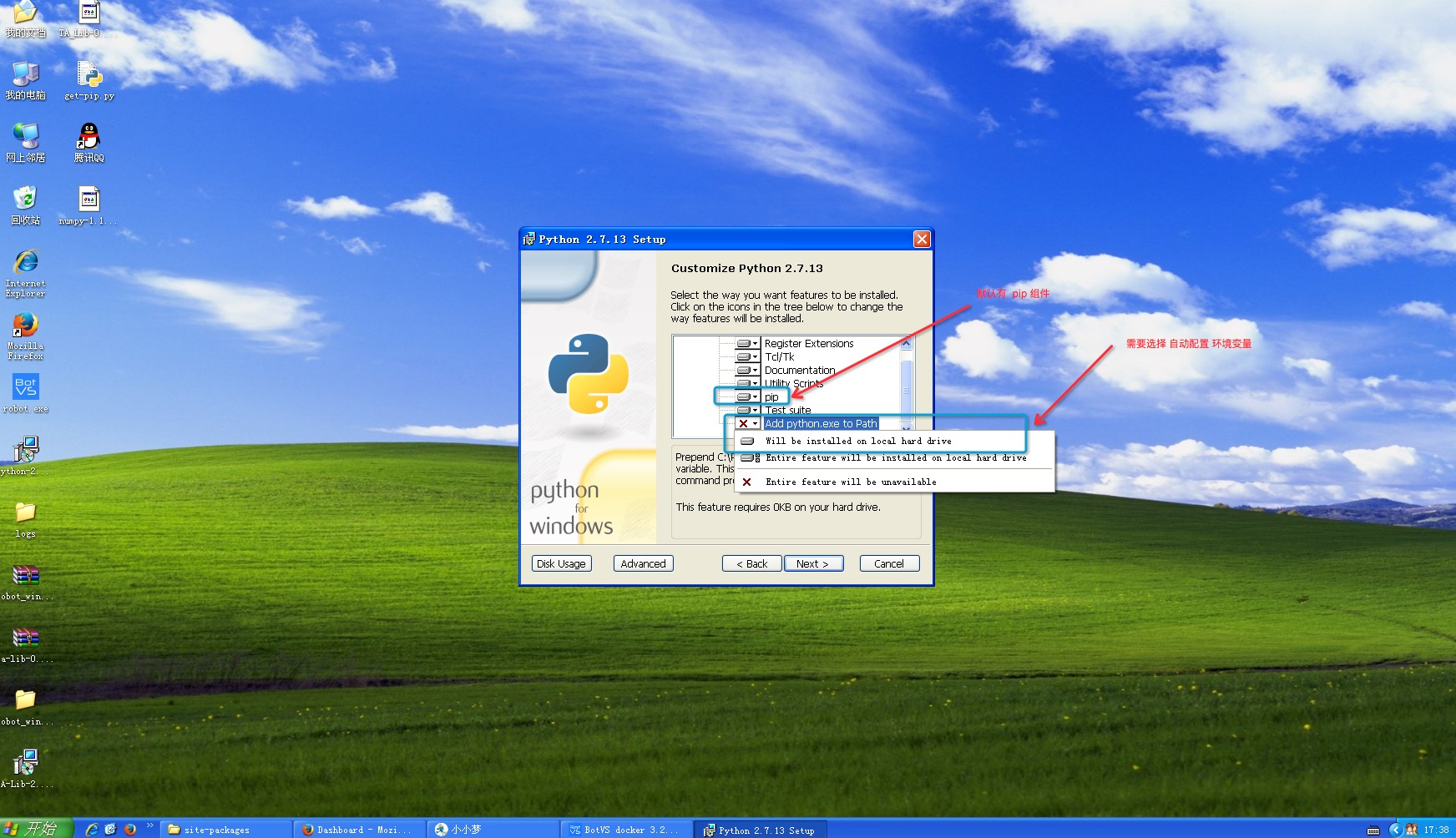• #### 2、安装 wheel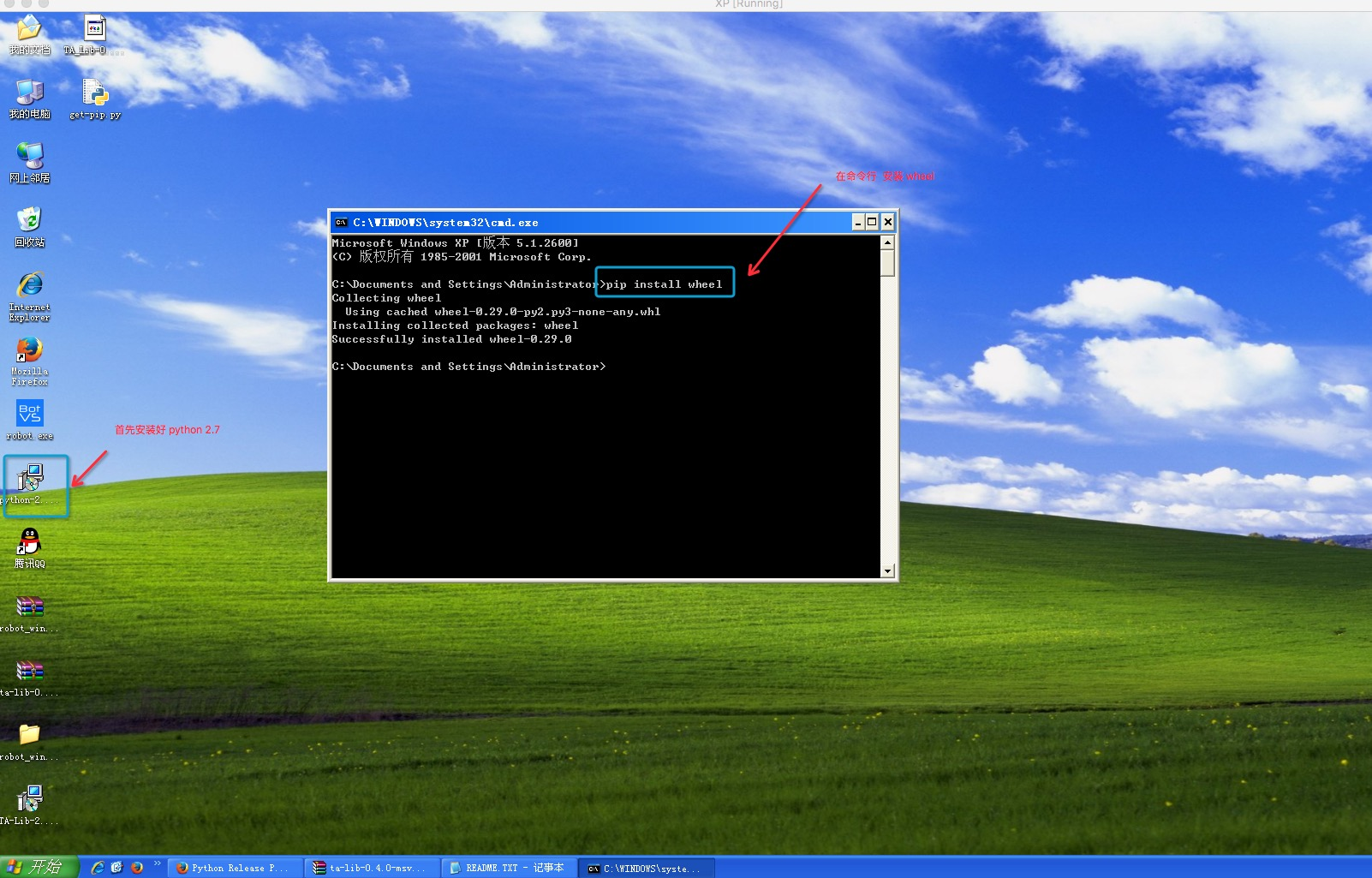以下是在网络上搜索的资料

``````python wheel怎么安装？

pip install wheel

pip freeze
``````
• #### 3、下载 talib 并安装

要找对应版本 和 系统 的 talib 文件 ， 如图：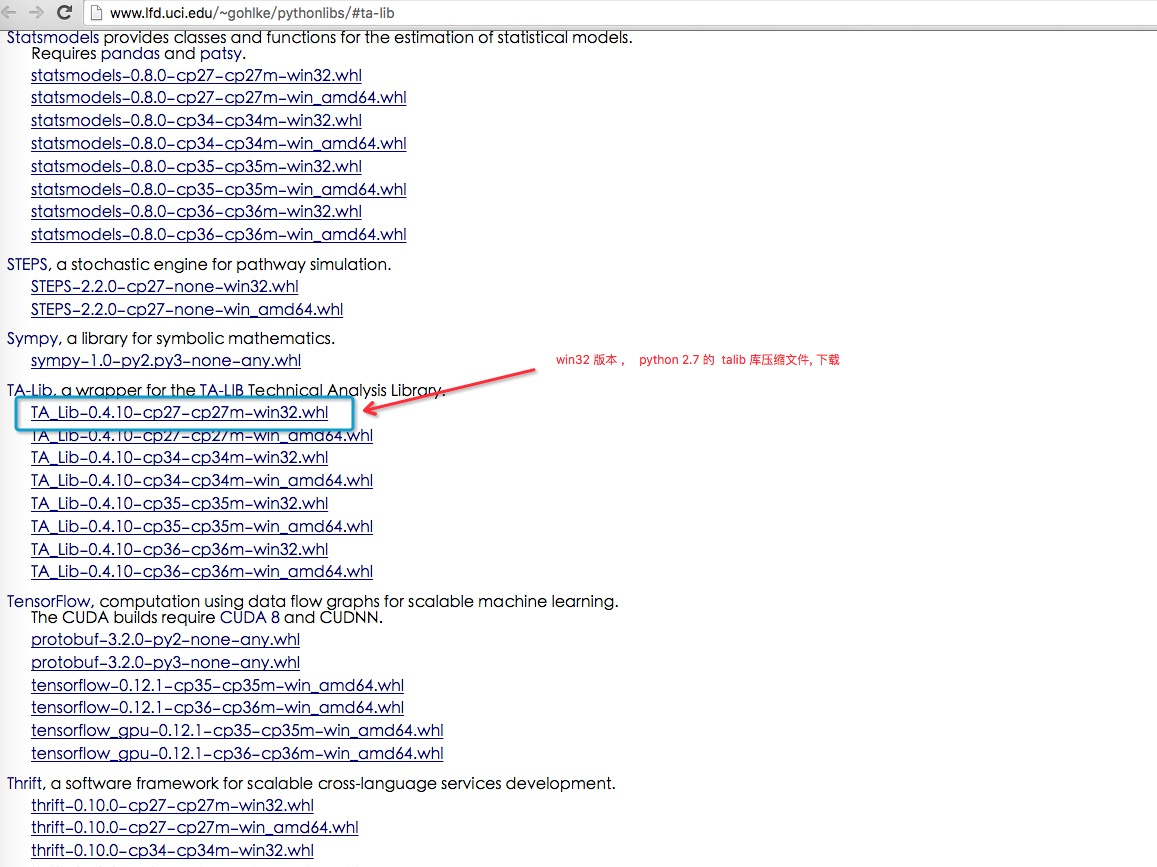下载好了以后 如下图 进行安装：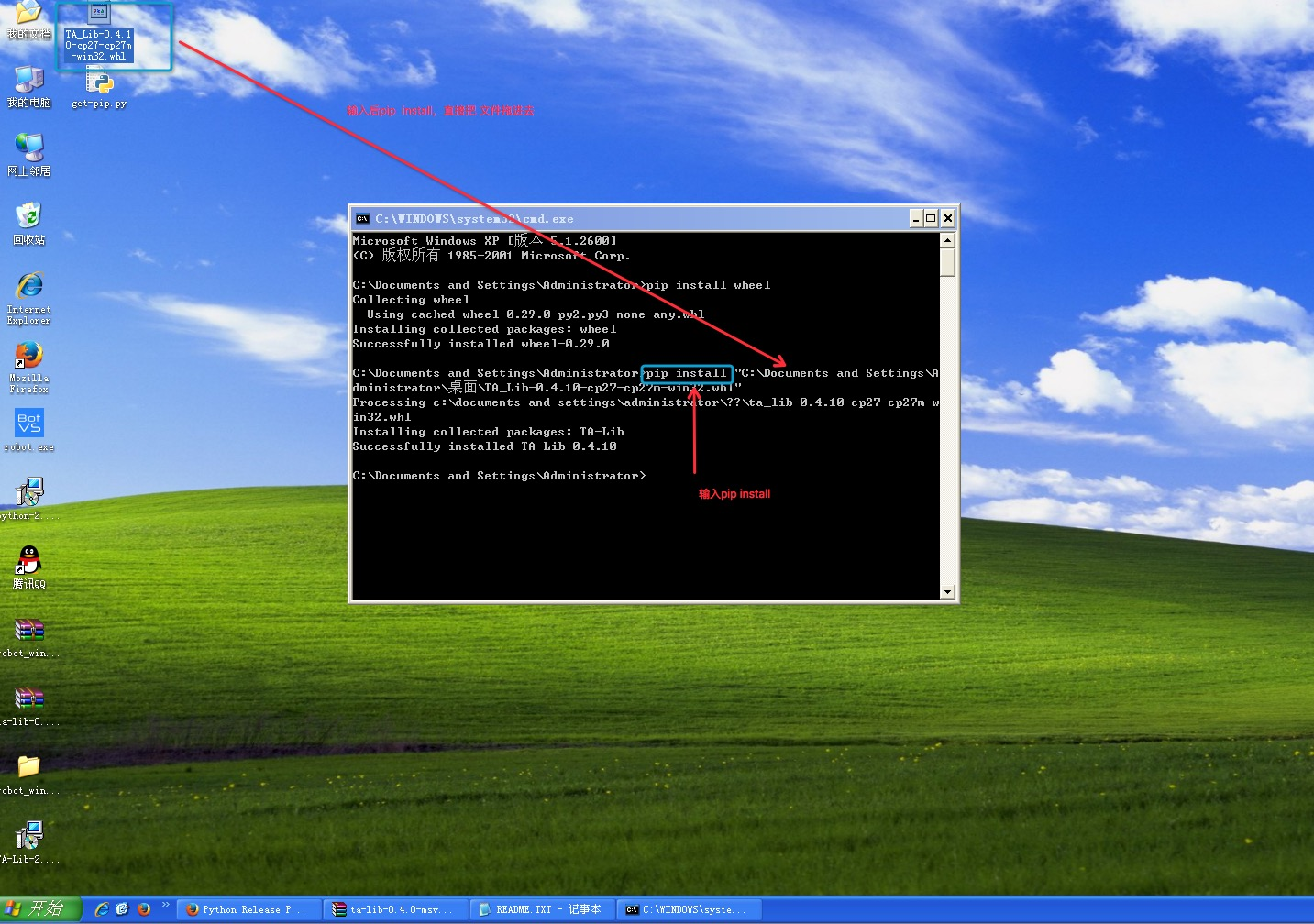• #### 4、本以为OK了 ，可是在使用 import talib 测试的时候 报错 显示 找不到 numpy , 看来这个 库 也要安装下。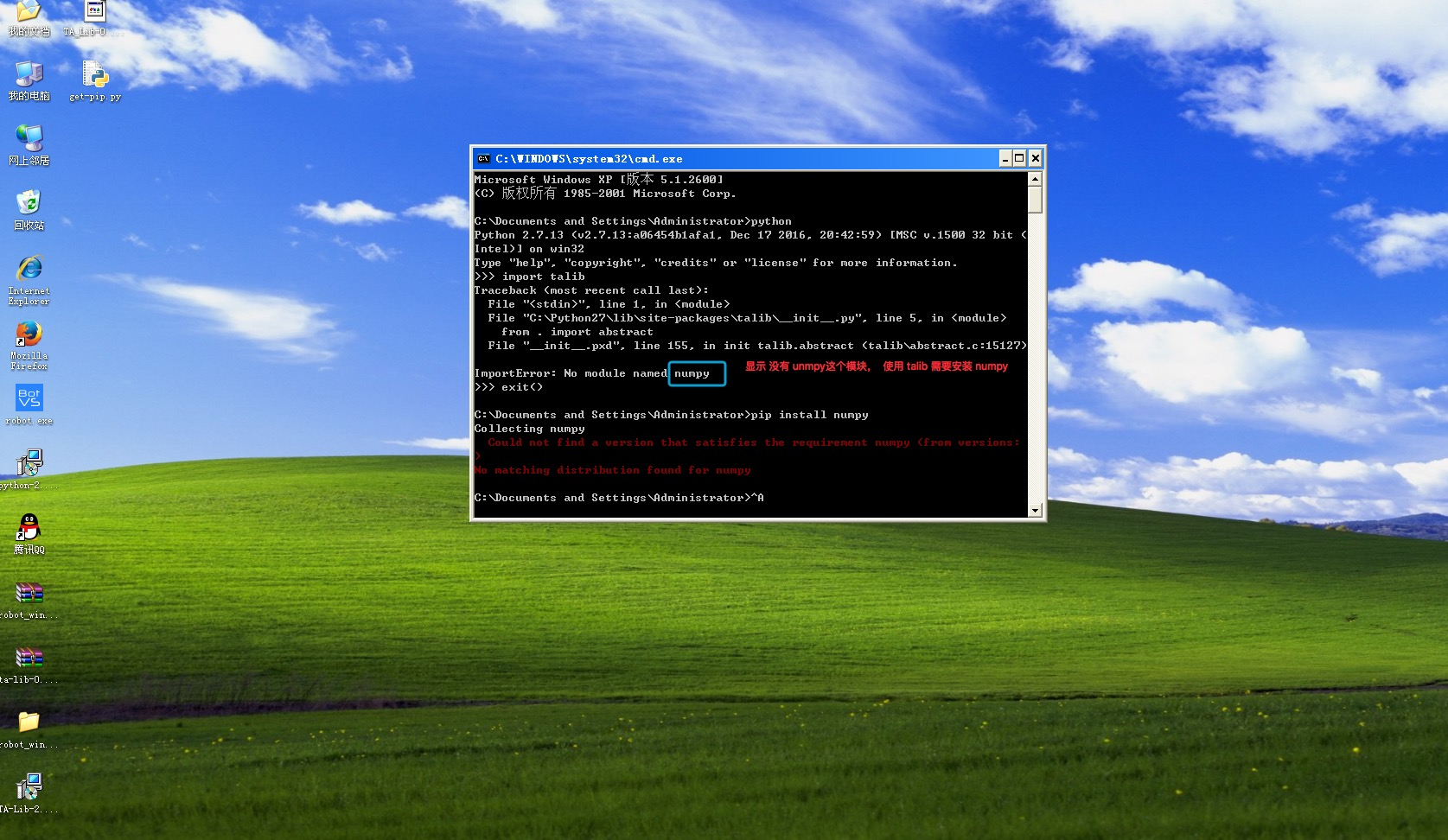下载 numpy 下载地址： http://www.lfd.uci.edu/~gohlke/pythonlibs/#ta-lib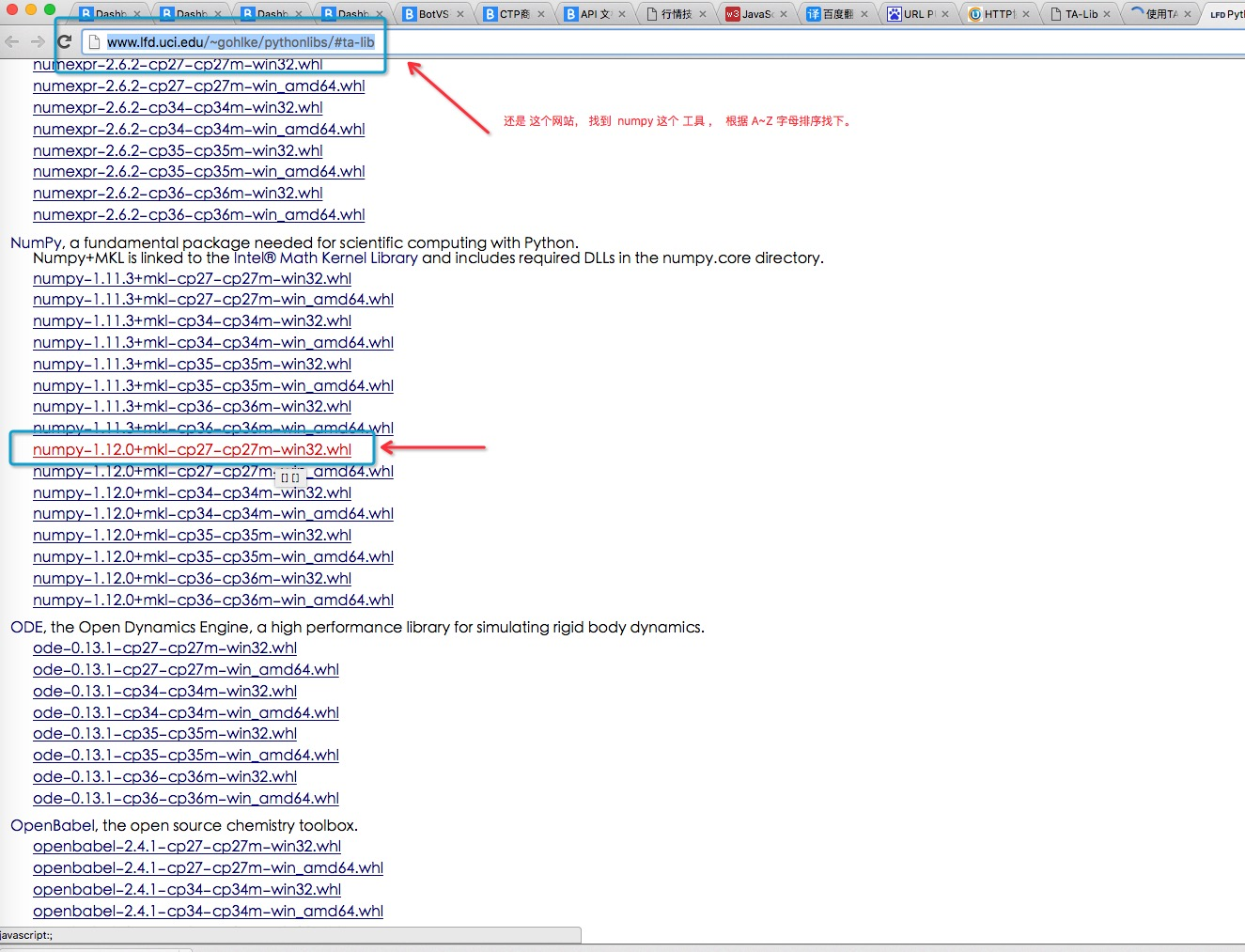安装：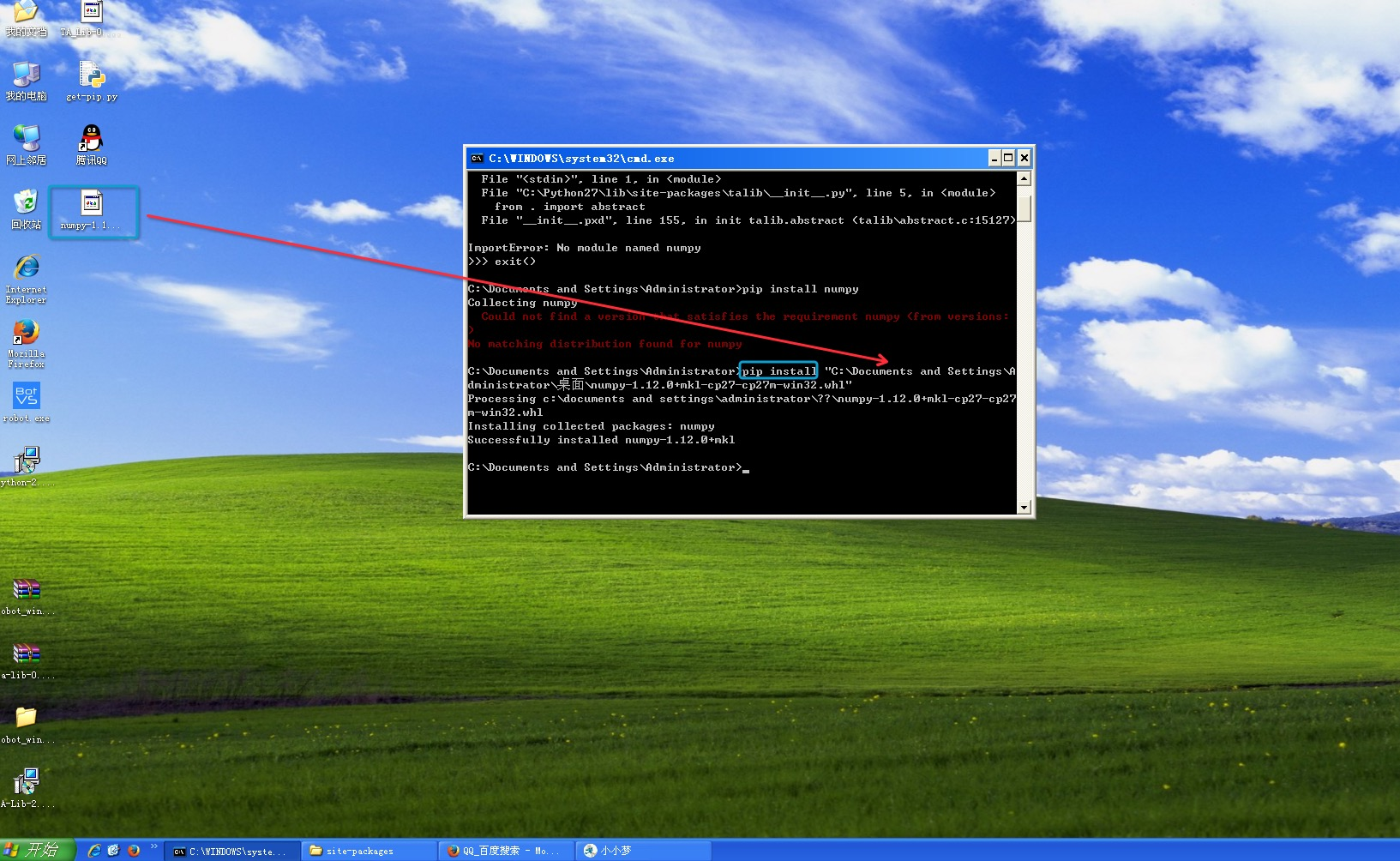• #### 5、测试一下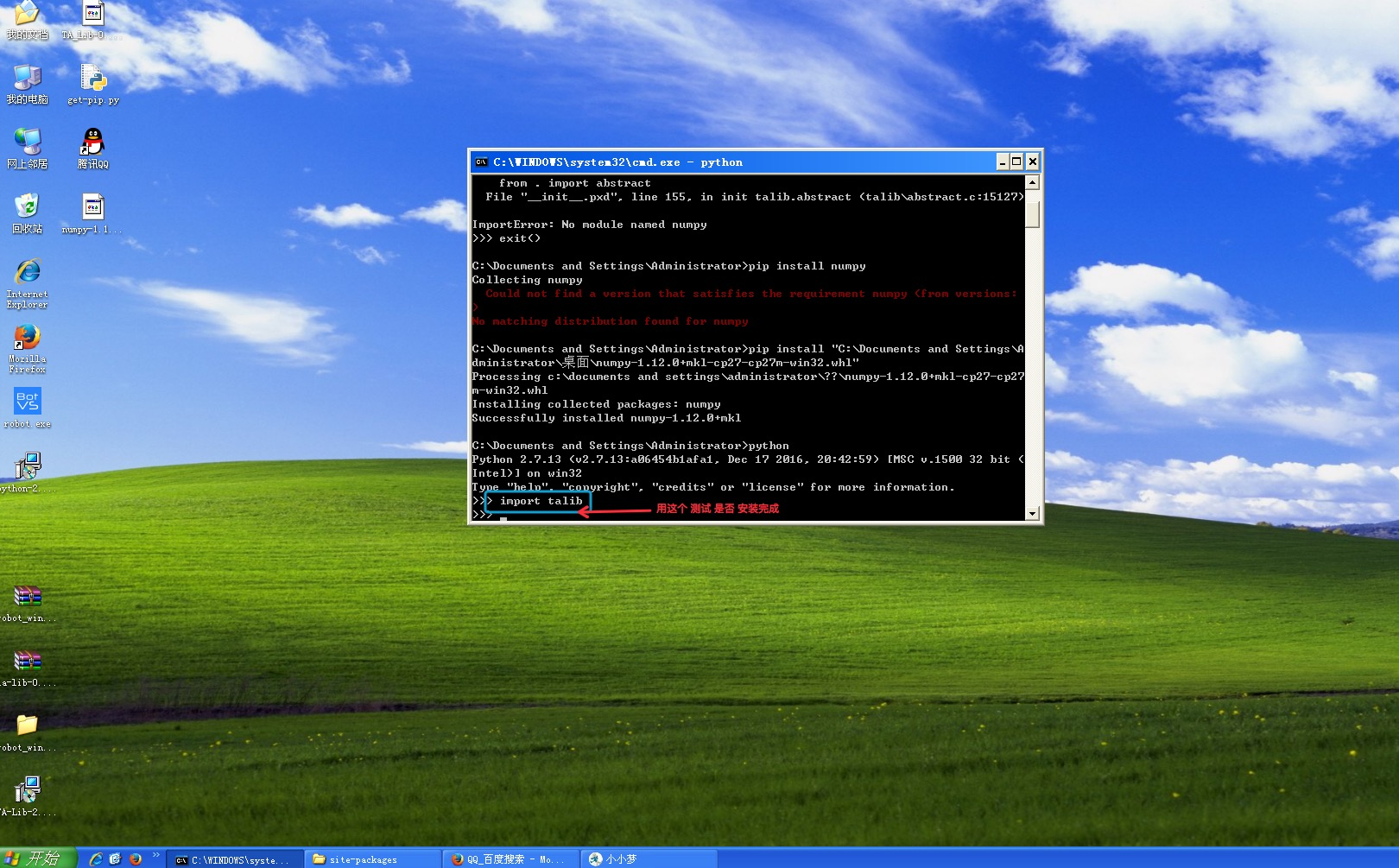#### 在 发明者量化 上试一下，策略使用 talib 的 指标函数

可以了 LogStatus 的输出显示出来了。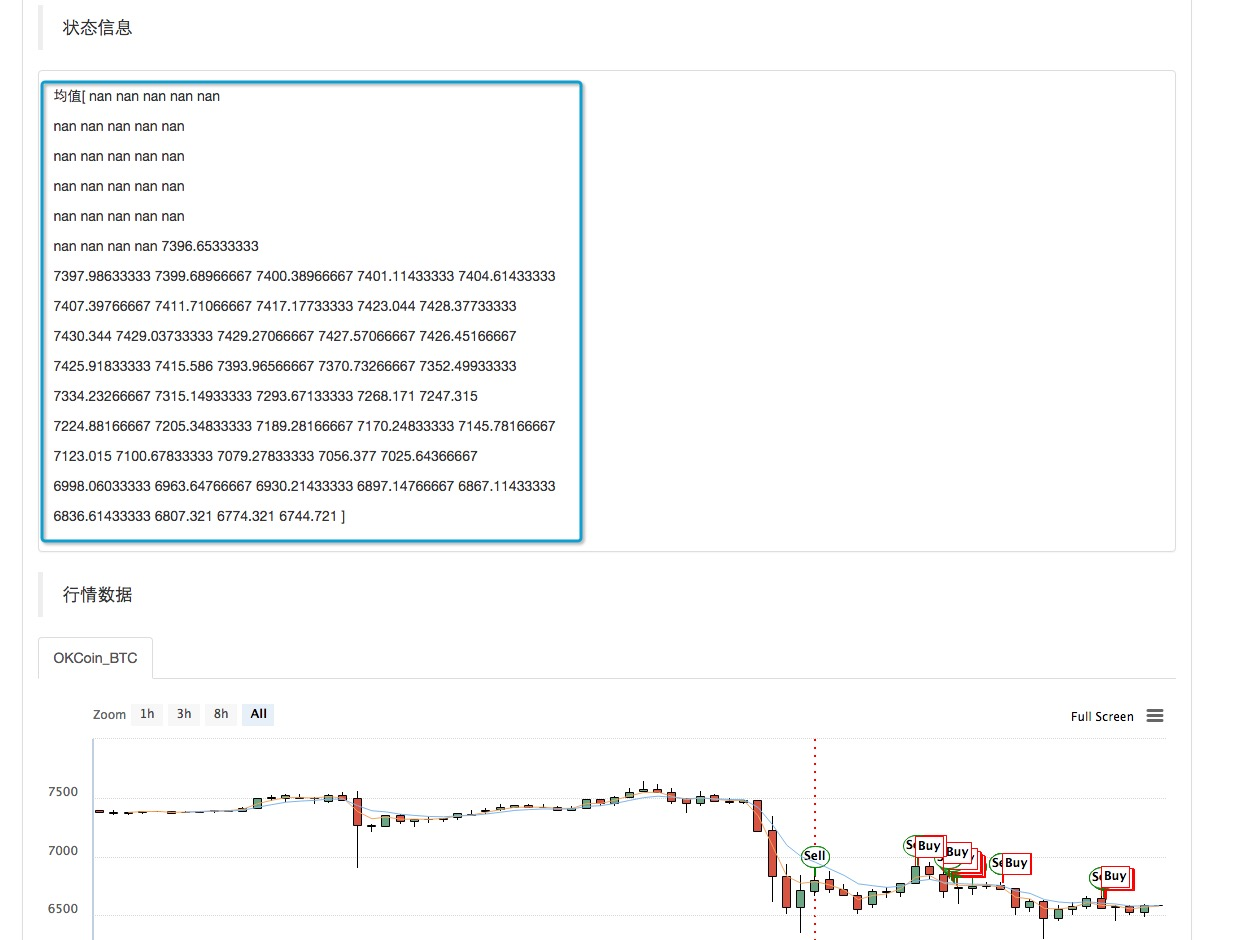• ### 以上过程中用到的 安装包 如下， 我已经传到QQ群。 用户也可以 按照教程地址 自行下载。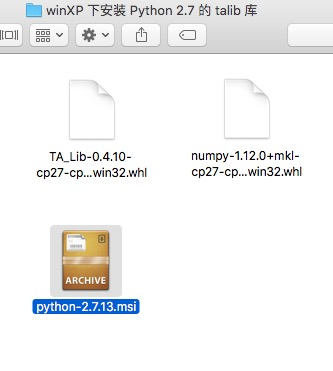压缩后More